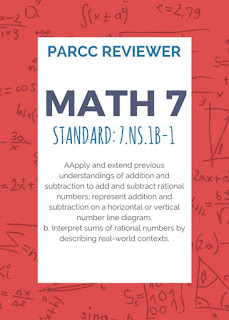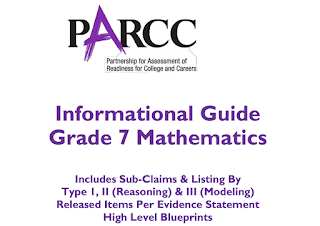## Labels

### Math 7 PARCC Reviewer 8 (7.NS.1b-1)

Sub-claim:
The students solve problems involving the Major Content with connections to the Standards for Mathematical Practice
Type of items: Type 1
Color Code: Peach
Evidence Statement Key: 7.NS.1b-1
Calculator: No
Point: 1 each (1:29)
Evidence Statement Text:
Apply and extend previous understandings of addition and subtraction to add and subtract rational numbers; represent addition and subtraction on a horizontal or vertical number line diagram. b. Understand p + q as the number located a distance |q| from p, in the positive or negative direction depending on whether q is positive or negative.
Clarifications, limits, emphases, and other information intended to ensure appropriate variety in tasks:
1. Tasks do not have a context.
2. Tasks are not limited to integers.
3. Tasks involve a number line.
4. Tasks do not require students to show in general that a number and its opposite have a sum of 0; for this aspect of 7.NS.1b-1, see 7.C.1.1 and 7.C.2.
Sample Questions (taken from PARCC’s Practice Tests and Released Items):
Practice Test, Unit 1, Item 9
2016 Released Item #91. Practice Test, Unit 1, Item 9

In which of these situations would the answer to the question be 0?
a. Teddy jumped into a pool from a diving board 8 feet above the water. He sank 8 feet and then swam straight up to the surface of the water. How many feet did Teddy swim?
b. Jerry left his house and walked 1.5 miles directly west. Then he walked 1.5 miles directly east. At this point, how many miles was Jerry from his house?
c. A trail begins at an elevation of -50 feet. The trail ends at an elevation of 50 feet. By how many feet does the elevation of the trail change from beginning to end?
d. The low temperature one day was -3° Celsius. The high temperature that day was 3° Celsius. What is the difference between the low temperature and the high temperature that day?

2. 2016 Released Item #9
Which number line demonstrates how to find the sum of 3 and -4?